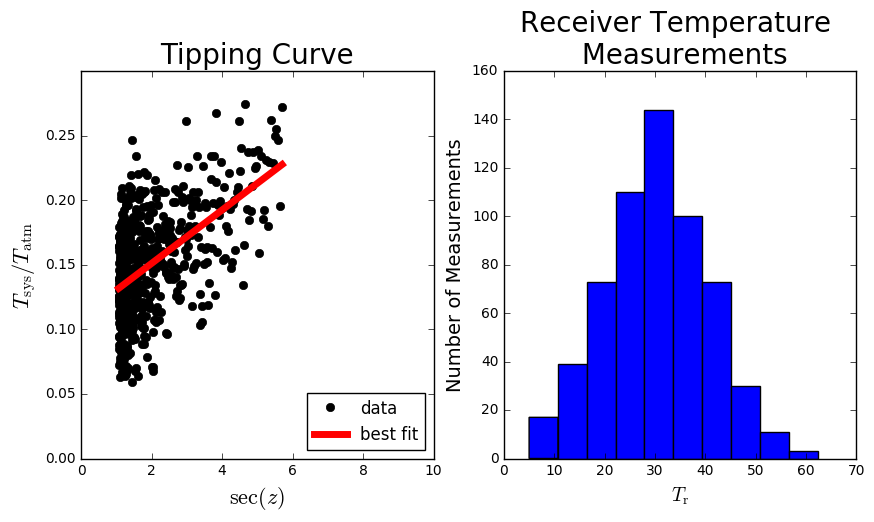# Worksheet 2: The Tipping Curve

This worksheet is based on Chapter 2 of the ERA Notes.

If the zenith opacity is sufficiently small, we can write the system temperature of a telescope as follows:

where $T_\mathrm{b}$, the equivalent temperature of the atmosphere, is given by $T_b=T_{atm} \tau_z \sec z$.

Since $T_\mathrm{r}$ and $T_\mathrm{cmb}$ are constants, we can thus write down the following expression for the tipping curve:

## Access

Construct a new Jupyter (IPython) Notebook using the commands below, and answer the following questions in your notebook.

# Questions

1. For each data set (measurement), use the data provided to estimate the system temperature and the temperature of the CMB.
2. For each measurement, calculate the RMS deviation of the receiver temperature $T_\mathrm{r}$. Now, plot the RMS deviations and the measured temperature and the calculated temperature $T_\mathrm{cmb}$. What can you conclude?

#### Tips & Assumptions

• You may assume that the ambient atmospheric temperature is measured to be $T_\mathrm{atm}=300K$.
• I have used a simple python library to fit a straight line to the tipping curve. This is a generic library to fit polynomials to data, and there is an associated function to calculate the resulting coefficients.
• The slope of the tipping curve is $[T_\mathrm{r}+T_\mathrm{cmb}]/T_\mathrm{atm}$, and the x-intercept is $\sec(z)$. Since the data is noisy, use a fit to estimate these parameters.
• The $T_\mathrm{r}$ receiver measurements resemble a Gaussian distribution. You can use this concept to estimate the mean value $% %]]>$, and the RMS deviation of the measurements.

The tipping curves are all located in the directory /data/ast2003h/, but you can also view and download them here.

You will find the following files in the /data/ast2003h/ directory:

In each file, column-1 corresponds to $\sec(z)$, column-2 corresponds to $T_\mathrm{sys}/T_\mathrm{atm}$ and column-3 corresponds to measurements of $T_\mathrm{r}$.

Note: In tipping-curve-example.txt there is an extra column-4, which corresponds to a fit to the tipping curve data. This is not present in the other files.

In the cells below I illustrate how to extract the data from tipping-curve-example.txt, and I plot the relevant data in two subplots. I’ve also plotted the best fit curve that I calculated previously.

import numpy as np
import pylab as pl
%matplotlib inline

data = pl.loadtxt('/data/ast2003h/tipping-curve-example.txt')
secz = data[:,0]
tsys_over_tatm = data[:,1]
trx = data[:,2]
fit = data[:,3]

pl.figure(figsize=(10,5))
pl.subplot(121)
pl.plot(secz, tsys_over_tatm, 'ko', label='data')
pl.plot(secz, fit, 'r-', lw=5, label='best fit')
# This sets up the x/y limits, axis labels and plot title.
pl.xlim(0,10)
pl.ylim(0,0.3)
pl.xlabel('$\sec(z)$', fontsize=16)
pl.ylabel('$T_\mathrm{sys}/T_\mathrm{atm}$', fontsize=16)
pl.legend(loc=4, numpoints=1)
pl.title('Tipping Curve', fontsize=20)

pl.subplot(122)
pl.hist(trx)
pl.xlabel('$T_\mathrm{r}$', fontsize=14)
pl.ylabel('Number of Measurements', fontsize=14)